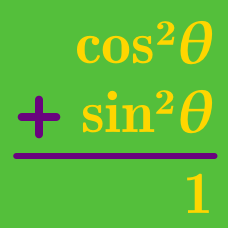Geometry

# Pythagorean Identities: Level 2 Challenges

Simplify: $\sqrt{1 - \sin^2 \theta}.$

$\large \frac { \sin ^{ 2 }{ \theta } }{ 5 } =\frac { \cos ^{ 2 }{ \theta } }{ 6 }$

If $\theta$ is a positive acute angle that satisfies the equation above, find $\sin { \theta }$.

Letting $P=\sin A \sin B \\ Q= \sin C \cos A \\ R = \sin A \cos B \\ S=\cos A \cos C$

Find the value of $5(P^2+Q^2+R^2+S^2)$ .

$\large\frac { 1 }{ \cos ^{ 2 }{ \theta } } +\frac { 1 }{ 1+\sin ^{ 2 }{ \theta } } +\frac { 2 }{ 1+\sin ^{ 4 }{ \theta } } +\frac { 4 }{ 1+\sin ^{ 8 }{ \theta } }$

If $\large \sin ^{ 16 }{ \theta } = \frac { 1 }{ 5 }$, what is the value of the expression above?

A triangle has sides of magnitude $1$, $\sin x$, and $\cos x$.

where $0 < x < \frac { \pi }{ 2 } .$

Find the largest angle of the triangle in degrees.

Assume that the triangle is non-degenerate.

×